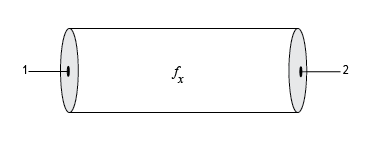# txlineEquationBased

Create equation-based transmission line

## Description

Use the `txlineEquationBased` object to create an equation-based transmission line. You can set the loss, the phase velocity, and the interpolation type in this object. You can also use the `txlineEquationBased` object to model an equation-based transmission line in an RF systems using an `rfbudget` object or the RF Budget Analyzer app.## Creation

### Syntax

``eqtxline = txlineEquationBased``
``eqtxline = txlineEquationBased(Name,Value)``

### Description

````eqtxline = txlineEquationBased` creates a default equation-based transmission line object.```

example

````eqtxline = txlineEquationBased(Name,Value)` sets Properties of the equation-based transmission line using one or more name-value arguments. For example, ```eqtxline = txlineEquationBased('Z0',75)``` creates an equation-based transmission line with the impedance of `75` ohms.```

## Properties

expand all

Name of the equation-based transmission line, specified as a string scalar or character vector.

Example: `'Name','EQbased'`

Example: `eqtxline.Name = 'EQbased'`

Operating frequency of the equation-based transmission line, specified as a positive scalar in Hz.

Example: `'Frequecny',2e9`

Example: `eqtxline.Frequency = 2e9`

Characteristic impedance of the equation-based transmission line, specified as a positive scalar in ohms.

Example: `'Z0',75`

Example: `eqtxline.Z0 = 75`

Loss of the equation-based transmission line, specified as a scalar in dB.

Example: `'LossDB',25`

Example: `eqtxline.LossDB = 25`

Phase velocity of the equation-based transmission line, specified as a positive scalar in m/sec.

Example: `'PhaseVelocity',2e9`

Example: `eqtxline.PhaseVelocity = 2e9`

Type of interpolation set in the equation-based transmission line, specified as `'Linear'`, `'Spline'`, or `'Cubic'`.

Example: `'IntType','Spline'`

Example: `eqtxline.IntType = 'Spline'`

Physical length of the equation-based transmission line, specified as a positive scalar in meters.

Example: `'LineLength',0.020`

Example: `eqtxline.LineLength = 0.020`

Stub transmission line termination in the equation-based transmission line, specified as `'NotApplicable'`, `'Open'`, or `'Short'`.

Example: `'Termination','Short'`

Example: `eqtxline.Termination = 'Short'`

Type of stub in the equation-based transmission line, specified as `'NotAStub'`, `'Series'`, or `'Shunt'`.

Example: `'StubMode','Series'`

Example: `eqtxline.StubMode = 'Series'`

Number of input and output ports in equation-based transmission line, returned as positive scalar.

Terminals of the equation-based transmission line, returned as a cell array of strings.

## Object Functions

 `sparameters` Calculate S-parameters for RF data, network, circuit, and matching network objects `groupdelay` Group delay of S-parameter object or RF filter object or RF Toolbox circuit object `noisefigure` Calculate noise figure of transmission lines, series RLC, and shunt RLC circuits

## Examples

collapse all

Create an equation-based transmission line `50` cm in length.

`eqtxline = txlineEquationBased('LineLength',0.05);`

Calculate the S-parameters of the equation-based transmission line over the frequency range `1``4` GHz.

```freq = linspace(1e9,4e9,51); sparam = sparameters(eqtxline, freq)```
```sparam = sparameters: S-parameters object NumPorts: 2 Frequencies: [51x1 double] Parameters: [2x2x51 double] Impedance: 50 rfparam(obj,i,j) returns S-parameter Sij ```

Calculate the group delay of the equation-based transmission line at `2.4` GHz.

` gd = groupdelay(eqtxline,2.4e9)`
```gd = 1.6678e-10 ```

## Version History

Introduced in R2021a